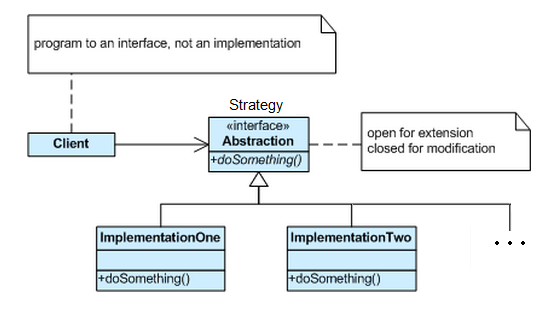# I need to get rid of that switch statement. What is the Strategy Pattern?

I’m a big advocate of software maintainability and there is nothing better for that than applying well known patterns to improve the existing code. Each time I see long if..then..else constructs, or switch statements to drive logic, I think of how much better the code would be if we allow encapsulation and use one of my favorite behavioral pattern… => the Strategy Pattern.A Strategy is a plan of action designed to achieve a specific goal

This is what this pattern will do for you: “Define a family of algorithms, encapsulate each one, and make them interchangeable. Strategy lets the algorithm vary independently from clients that use it.” (Gang of Four);

Specifies a set of classes, each representing a potential behaviour. Switching between those classes changes the application behavior. (the Strategy). This behavior can be selected at runtime (using polymorphism) or design time. It captures the abstraction in an interface, bury implementation details in derived classes.

When we have a set of similar algorithms and its need to switch between them in different parts of the application. With Strategy Pattern is possible to avoid ifs and ease maintenance;

Now, how can we digest that in code, now that you got the gist of the problem and want a better solution than your case statements.

This example I’ll be showing is a pure academic exercise:

The problem to solve is given a string as an input, create a parsing algorithm(s) that given a text stream identifies if the text complies with the following patterns. Angle brackets should have an opening and closing bracket and curly brackets should also have an opening and closing bracket, no matter how many characters are in the middle. These algorithms must be tested for performance.

```<<>>{}  True
<<{>>}  False
<<<>    False
<<erertjgrh>>{sgsdgf} True

```

``` ```   public interface IStringValidator
{
bool Validate(string input);
}

public class StackValidator : IStringValidator
{
public bool Validate(string input)
{
Stack<char> fifo = new Stack<char>();

if (input.Length == 0) //all characters were nicely paired with no chars in between
{
return true;
}
else if (input.Length == 1)
{
return false;
}
else
{  //if the input has more characters or the pairs  have  different characters in between

char[] cArr = input.ToCharArray();
foreach (char c in cArr)
{
if ((c == '<') || (c == '{'))
{
fifo.Push(c);
}
if ((c == '>') || (c == '}'))
{
if (fifo.Count == 0)
{
return false; //no start parentheses < or { was pushed to stack, stack is empty, however a closing character was found, thus false
}

char top = fifo.Peek();
if ((c == '>') && (top == '<'))
{
//found a pair
fifo.Pop();
}
else if ((c == '}') && (top == '{'))
{
//found a pair
fifo.Pop();
}

}
}
if (fifo.Count == 0)
return true;
else
return false; //the stack has < and { characters that never matched a closing char
}
}
}

public class RegexValidator : IStringValidator
{
public bool Validate(string input)
{

Regex exp = new Regex(@"(<(\w*\d*\s*{?}?)([^{}<>])*>)|{((\w*\d*\s*<?>?)[^{}<>])*}");

while (exp.IsMatch(input))
{
MatchCollection m = exp.Matches(input);
foreach (Match n in m)
{
input = input.Replace(n.Value, string.Empty);

}
}

if (input.Length == 0) //all characters were nicely paired with other chars in between
{

return true;
}
else
{
return false;
}
}
}```
```

What would be calling this code? For simplicity purposes, a Console C# program, here it goes:

```class Program
{
static void Main(string[] args)
{

string[] arr = Initialize();

long av1 = ProcessStrings(arr, new StackValidator());

long av2 = ProcessStrings(arr, new RegexValidator());

int j = arr.Length;

Console.WriteLine("Average for Stack algorithm {0}, total {1}", (av1 /j),av1);
Console.WriteLine("Average for Regex only algorithm {0}, total {1}", (av2 / j), av2);